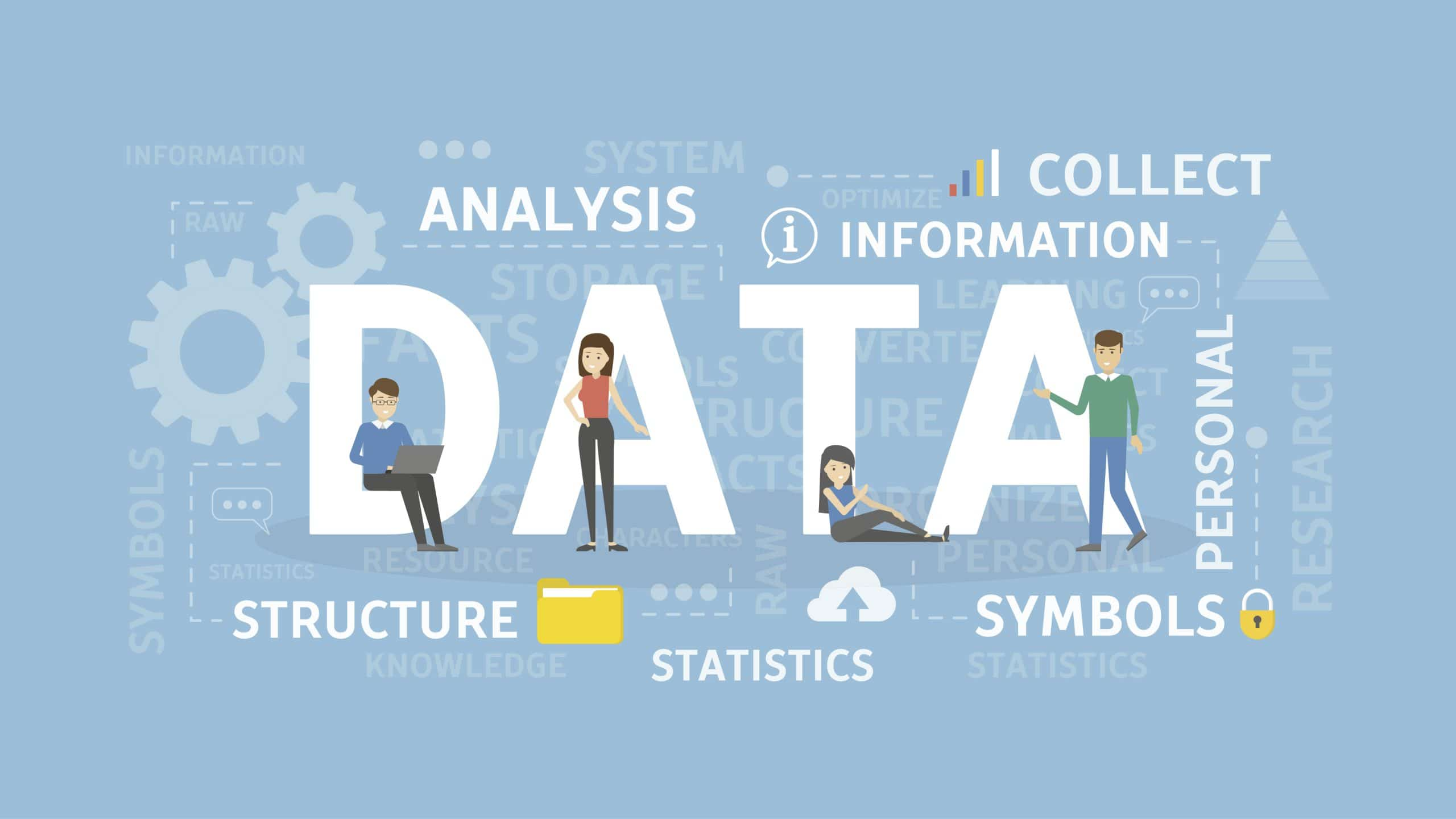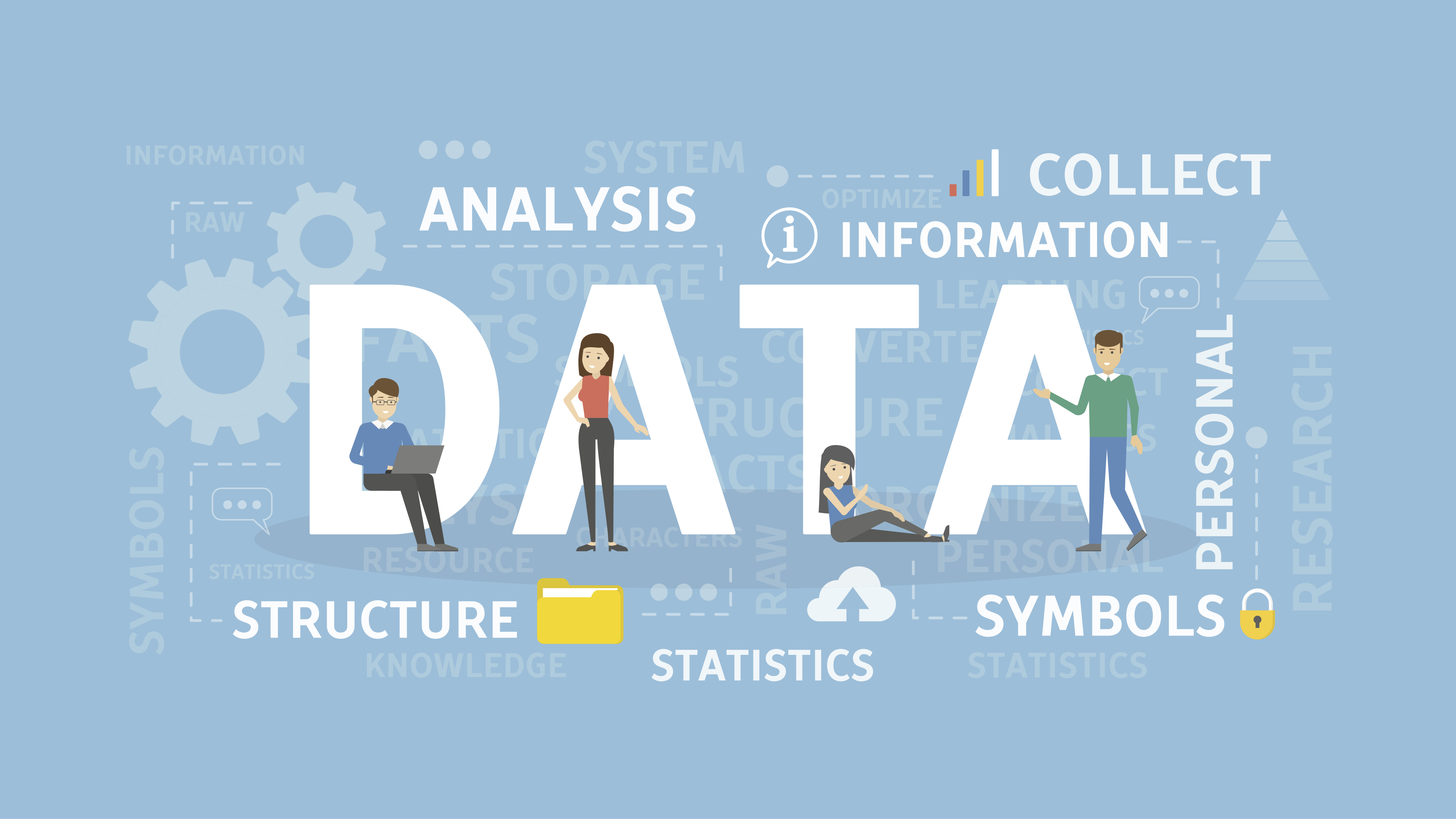# Data Science 101: An Introduction into the World of Predictive Analytics

Welcome to data science 101, your guide to important introductory topics in predictive analytics. If you are an analytics specialist looking to learn more about data science, or even just someone interested in learning more about the topic, then this is the series for you!We want to show you that if you do not have a strong background in statistics, or if you are not considering yourself as a data scientist, you can still effectively work with and analyze data. Throughout this four part series, we will go through the following topics:

Throughout this series we will use a real data set to help explain and demonstrate the discussed concepts. Should you choose to follow along with us, we have all of our work available to you in the following links:

• For Python gurus, click HERE to download a zip file that contains the Jupyter Notebook and necessary csv files. When in Jupyter Notebook, only open the file “Blog Post Data Set and Tests (Code).ipynb” to access the notebook with the code. The other files within the folder are just csv files of the data to be read in the notebook.

All of the calculations were done in both Excel and Jupyter Notebook, but all of our explanations are done using Excel. The Jupyter Notebook file will show you how we coded the same tests as well as the graphics and figures using Python.

Before jumping into any tests or analysis, we first go through how to approach your raw data set. We have a lot to get through, so let’s get started!

The Basics: How to Look at Your Data

Before we even begin to analyze our data, it is important to first assess our raw data. Ensuring you have an in depth understanding of your data allows you to better manipulate it and draw better conclusions. When collecting or selecting data, it is important to keep two overarching questions in mind:

1. Who is my target audience?
2. What question am I trying to answer?

When thinking of your target audience, you want to make sure that your data is applicable and appropriate for that population. For example, if you were exploring housing prices for an intended US audience, it would be more beneficial to find data for houses in the United States rather than in Europe or Canada. When thinking of your question, you must ensure that your data is actually is viable for answering it. Think about the type of data you are looking for, whether it be qualitative or quantitative. For example, if you were looking at tumor sizes and dimensions, quantitative data would be more advantageous to use, but if you were looking at tumor attributes, such as shape and texture, qualitative data would be better to look at. In addition, it is important to gather any and all information that you may have about the data set. For example:

1. Where was the data collected from?
2. Who collected the data?
3. When was the data collected?
4. How large is the data set?

Some of these questions may be more relevant than others, especially depending on whether your data is primary, meaning it was collected yourself firsthand, or secondary, meaning it was collected by someone else that you intend to use secondhand. The goal of data analytics is to answer a question, and that begins with information outside of just the numbers.

Throughout this series, we will apply these thought processes and methods to a real, secondary data set pertaining to housing prices. This data set contains a sample of 21,613 houses describing their price, number of bedrooms, number of bathrooms, number of floors, grade, condition, size of the living room, size of lot, square footage of the house above ground level, size of the basement, the year it was renovated, and the year it was built. The data was collected from King County in Washington in 2015.

We are looking to answer the question: what characteristics of a home affect its price? This is an extremely overarching question that will require multiple methodologies to reap the answers we want. In the next section, we will briefly discuss key statistical terms and methodologies so you can better understand the proceeding content.

Key Statistical Terms and Definitions

Before we dive in, let’s talk definitions. Just like any other field of study, statistics has its own language, or jargon. If you aren’t a native statistics speaker, understanding this language may seem a little bit confusing, but hang with us! Throughout the series we will ensure that you can decode some of the tricky statistics language that we use, starting with this brief section where we define some critical terms and topics that you will hear frequently throughout. If you ever get confused in latter sections, just pop back up here and read through our explanations. Here is a list of some of the important terms we will be using throughout this series:

• Primary Data → Data that has been collected by you, firsthand
• Ex: Surveys, interviews, experiments, focus groups, etc.
•  Secondary Data → Data that has been collected or produced by someone else and used secondhand by another person other than the researcher
• Ex: Information libraries, public government data, population census, etc.
• Statistical Significance → In essence, this just means that something is of importance and worth investigating. If something is statistically significant, to be a bit more technical, that means that the relationship between two variables is likely not due to chance alone and can be attributed to something else.
• Practical Significance → Tells us how applicable or relevant our findings actually are; shows the magnitude of our statistical significance
• P-value → p-value, or probability value, is possibly one of the most important statistical terms to be discussed. This metric places a numerical value on statistical significance. There are different p-value cut-offs that can be used, but it is standard to say that a p-value less than or equal to 0.05 indicates statistical significance (we will be using this cutoff throughout the remainder of the series).
• Discrete Variable → A variable that can only take on a finite number of values
•  Ex: Number of rooms, number of floors, number of pets
• Continuous Variable → A variable that can take on an infinite number of values
•  Ex: Height, square footage of a house, weight
• Hypothesis Testing → Just like a science experiment, in hypothesis testing, we are simply trying to test a hypothesis or a “prediction” that we may have. In hypothesis testing, we will form two types of hypotheses:
• Null Hypothesis → Put simply, this is our hypothesis, or statement, that there is nothing going on between our two variables.
•  Ex: There is no statistically significant difference between the price of pencils at Target and the price of pencils at Walmart
• Alternative Hypothesis → This is the claim that we are trying to prove with the hypothesis test; once again, put simply, this means that there is, in fact, something going on between our two variables worth noting
•  Ex: There is a statistically significant difference between the price of pencils at Target and the price of pencils at Walmart
•  Note that all hypothesis testing is done under the assumption that the null hypothesis is true
• Dependent Variable → Also known as the “y” variable
• Independent Variable → Also known as the “x” variable; the variable we think has some effect on the dependent variable
• Linear Regression → This is a commonly used methodology to see if one variable can be used to predict another. There are two types of modeling methods:
• Single Linear Regression → Seeing if one, independent variable, or predictor, is good at predicting a dependent variable
•  Ex: Is an SAT score a good predictor of GPA?
• Multiple Linear Regression → Seeing if multiple independent variables / predictors have a relationship with a dependent variable
•  Ex: Are AP, SAT, and ACT scores good predictors of GPA?
• Correlation → The relationship between variables, typically denoted as r; r is between -1 and 1, with -1 being perfectly, negatively correlated and 1 being perfectly, positively correlated. The closer the r value is to |1|, the stronger the association. If your r value is 0, that means that there is absolutely no correlation between the two variables, so the closer the r value is to 0, the weaker the association.

If you’re ever confused about certain terminology used throughout, you can jump back up here for a quick refresher!

Can’t get enough? Well neither can we! In our next installment of this series, we will dive into the importance of the distribution curve and sample size, two concepts that are imperative for setting the stage for most of our consequent statistical testing. Click here to read.## Stay in Touch

Get the latest news, posts, and in-depth articles from dbSeer in your inbox.

This field is for validation purposes and should be left unchanged.